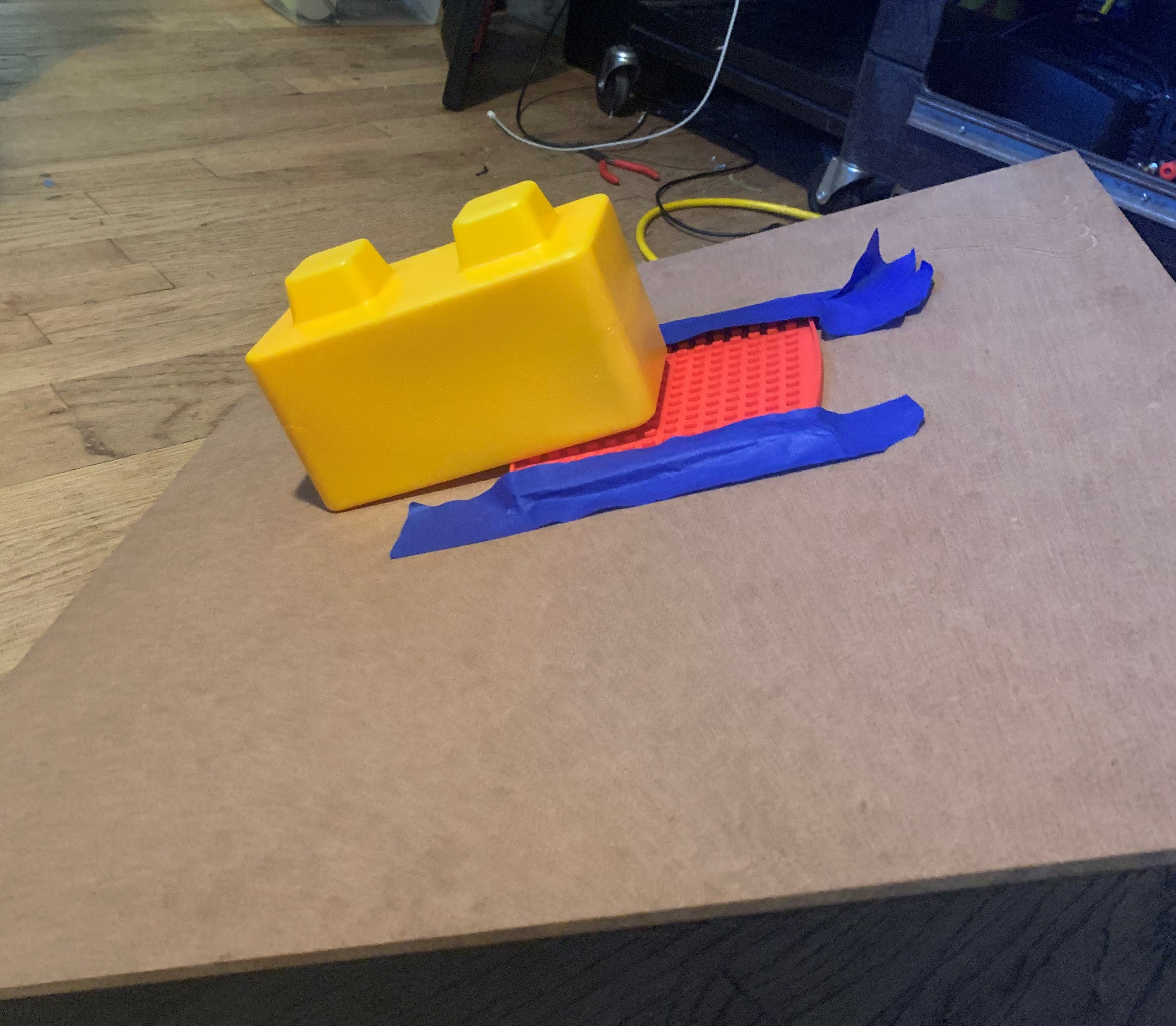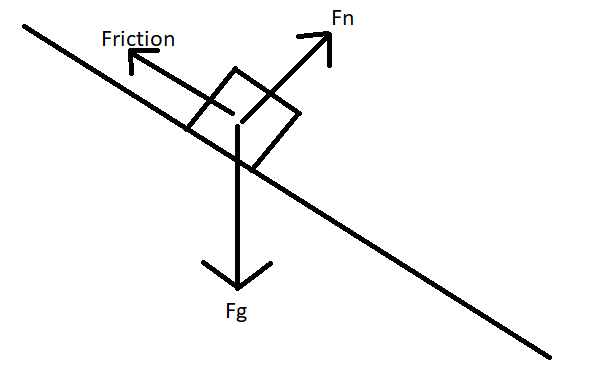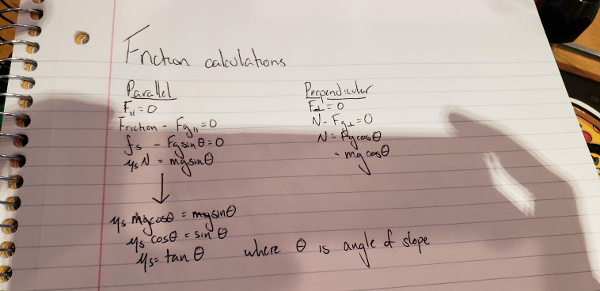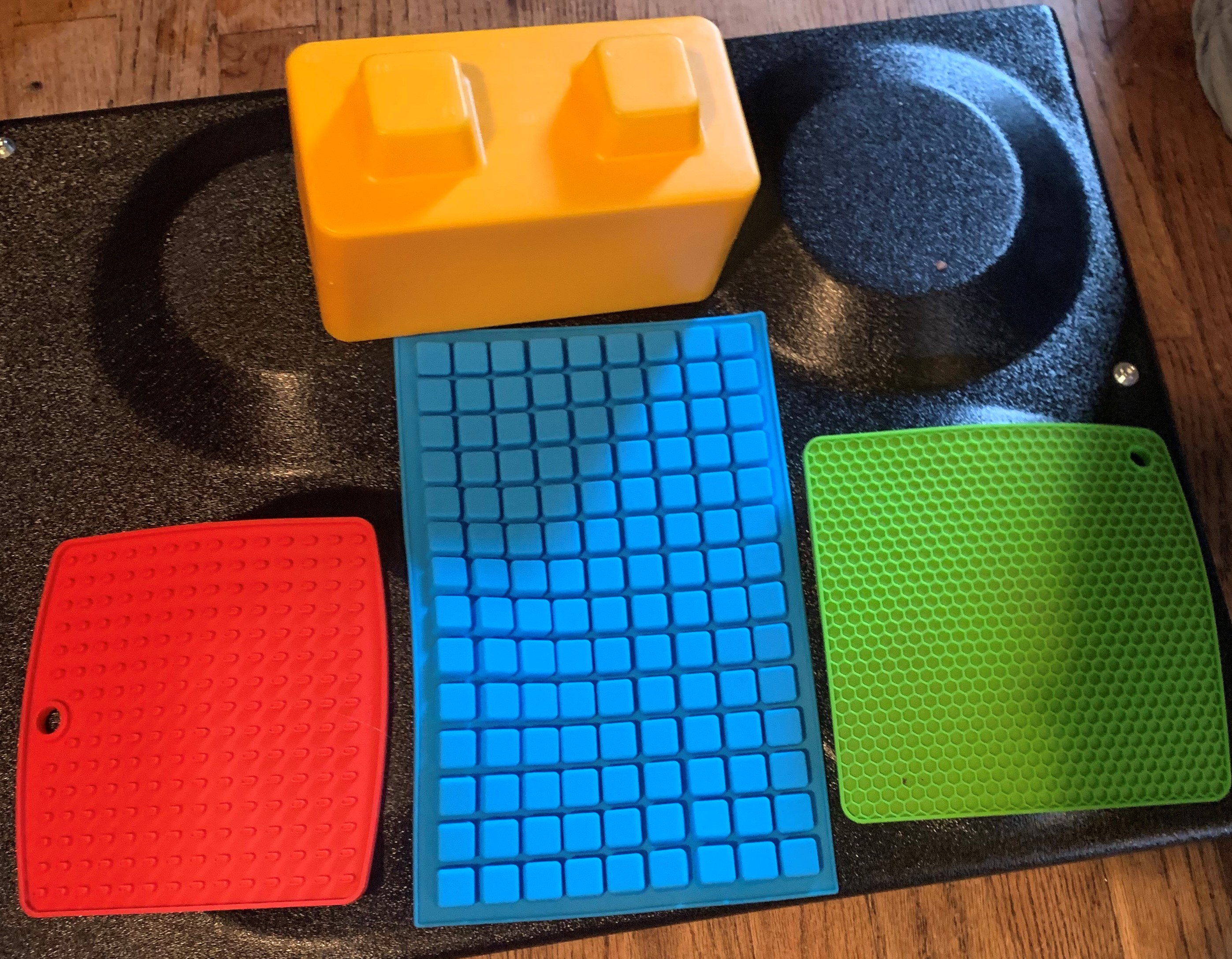# Testing Friction Coefficient

Tags: think and design
Personhours: 4
Testing Friction Coefficient By Bhanaviya

### Task: Measure the coefficient of friction of our potential gripper materialsWe want to measure various constants of materials on our robot. These materials serve to improve the grip on our gripper. But before we can decide which material will be most effective on our gripper, we need to find the friction coefficient of these materials through a slip test. The slip test is detailed in a separate post in E-67. This article serves mainly to show the specific friction coefficient produced by each material in the slip test.To measure the coefficient of friction, we first had to simplify an equation to determine what values to measure.Based on these calculations, we realized that the best way to calculate friction coefficient would be by deriving the angle of incline at which the skystone begins to slip from the material, which is placed on a flat wooden board. If we take the length of the side of the board being lifted to be the hypotenuse, and the height at which the board is being lifted to be height, then theta, the angle of incline, is arcsin. As the board is lifted, the stone begins to experience slippage and the angle at which it slides off the material being tested will be marked as its friction coefficient. The higher this value, the more grip the material has.The materials we will be testing are a green silicon oven-mitt with hexagonal ridges, a red silicon oven mitt with small rectangular ridges, and an ice-cube tray with cubical ridges. The wooden board on which this the materials and skystone are being placed on has a length and width of 23.5 inches. This will be the hypotenuse for the purposes of this test.

#### Green Silicon Oven-Mitt

When the wooden board was lifted with this material on top of it, it took a height of 10.3 inches before the stone began to slip. Using arcsin, the angle of incline for this material was 26 degrees. By using the equation above, we can find that the coefficient of friction is tan(26) which 0.48.

#### Red Silicon Oven-Mitt

When this material was tested, the board had to be lifted 13.7 inches before the stone began to slip. The angle of incline for this material was 35 degrees so the coefficient of friction is tan(35) which is 0.7. Since this value is higher than the green oven-mitt, the red oven-mitt has the better grip.

#### Ice-Cube Tray

The board reached a height of 12.2 inches when the stone began to slip from the ice-cube tray. The friction coefficient for this material was 32 degrees, and its coefficient of friction was hence 0.66, putting it above the green oven-mitt, and slightly below the red oven-mitt in grip.

### Next Steps

The material with the largest friction coefficient will be attached to the gripper on the robot. Since the red silicon oven-mitt had the largest angle of incline, this will be the material we will use in the next iteration of our gripper.

Date | December 28, 2019